1. EMS: Emergency Medical Systems

1) Main Theme

• “Ambulances deliver patients to hospitals.”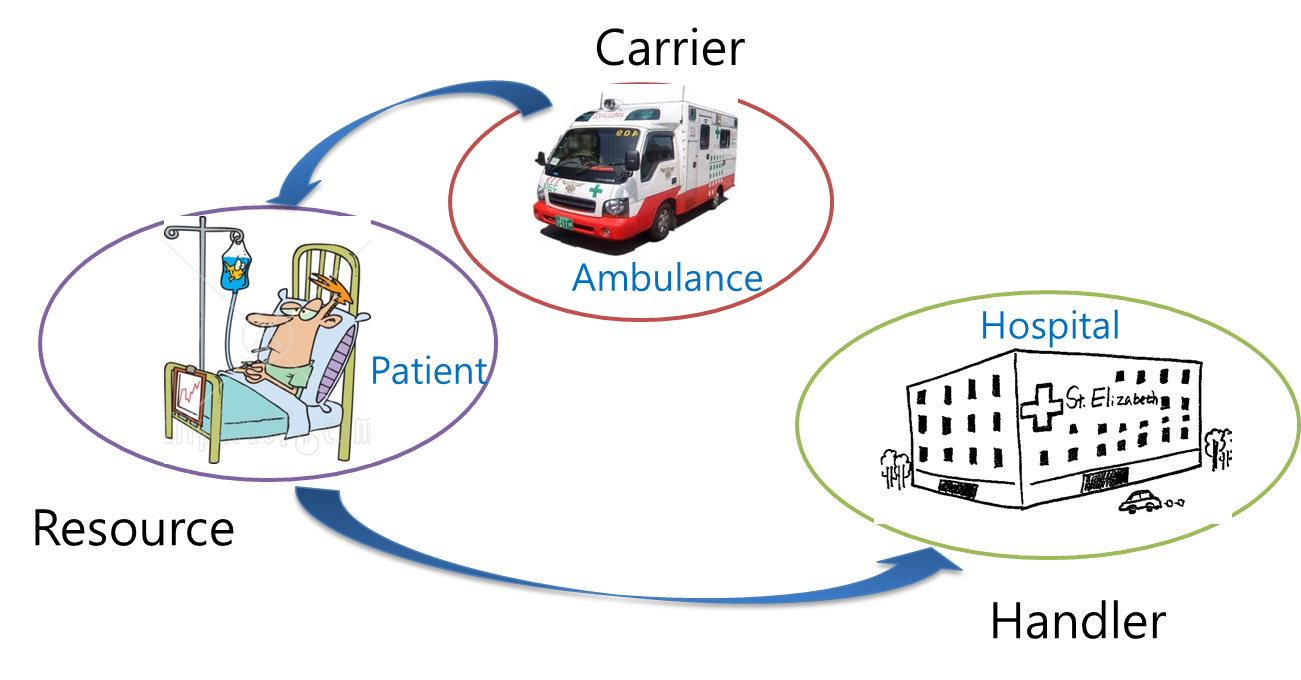2) Configuration

• Carrier: 2 Ambulances
• A, B in 911 Center
• Sources: 5 Places
• 2 Houses: A, B
• Resources: 4 Patients
• 2 Patient at House A
• 2 Patient at House B.
• Destination: 3 Hospitals
• A: 2 rooms
• B: 2 rooms

2. Operational Requirements

• One of the patients at each location calls 911 of their status.
• The 911 sends its ambulance to the location.
• Each ambulance moves to the location to transport the patients  to hospitals.
• The ambulance follows orders from 911.
• Once two patients at the location get on the ambulance, the ambulance moves to the hospital.
• Each patient will be transported to the appropriate hospital for treatment.
• Once all the patients are properly transported, all the ambulance will return to the 911.

3. Specification: d-Calculus

• EMS=911[AM1∥AM2]∥HA∥HB∥L1[PA1∥PB1]∥L2[PA2∥PB2]
• 911=P(L1 Info).A1(R ̅ ).AM1 out.A1((L1) ̅ ).P(L2 Info).A2(R ̅ ).AM2 out.A2((L2) ̅ ).
• ((A1((getAB) ̅ ).A2((getAB) ̅ )) ⨁_1^1 ((A1((getA) ̅ ).A2((getB) ̅ )) ⨁_2^2 (A1((getB) ̅ ).A2((getA) ̅ )))). AM1 in.AM2 in.exit
• AM1=A(R).out 911.A1(L1).(A1(getAB) ⨁_1^1 (A1(getA) ⨁_2^2 A1(getB))).
•  ((in L1.get PA1.get PB1.out L1) ⨁_1^  ((in L1.get PA1.out L1) ⨁_2^  (in L1.get PB1. out L1))).
•  ((in HA.put PA1.out HA) ⨁_1^  ((in L2.get PA2.out L2) ⨁_2^  (in L2.get PB2.out L2))).
•  ((in HB.put PB1.out HB) ⨁_1^  ((in HA.put PA1.put PA2.out HA) ⨁_2^  (in HB.put PB1.put PB2.out HB))).
•  in 911.exit
• AM2=A(R).out 911.A2(L2).(A2(getAB) ⨁_1^1 (A2(getB) ⨁_2^2 A2(getA))).
•  ((in L2.get PA2.get PB2.out L2) ⨁_1^  ((in L2.get PB2.out L2) ⨁_2^  (in L2.get PA2. out L2))).
•  ((in HB.put PB2.out HB) ⨁_1^  ((in L1.get PB1.out L1) ⨁_2^  (in L1.get PA1.out L1))).
•  ((in HA.put PA2.out HA) ⨁_1^  ((in HB.put PB1.put PB2.out HB) ⨁_2^  (in HA.put PA1.put PA2.out HA))).
•  in 911.exit
• L1=((AM1 in.AM1 out) ⨁_^1 (AM1 in.AM1 out.AM2 in.AM2 out)).∅^∞
• L2=((AM2 in.AM2 out) ⨁_^1 (AM2 in.AM2 out.AM1 in.AM1 out)).∅^∞
• PA1=P((L1 Info) ̅ ).((AM1 get.AM1 put) ⨁_^1 ((AM1 get.AM1 put) ⨁_^2 (AM2 get.AM2 put))).∅^∞
• PB1=((AM1 get.AM1 put) ⨁_^1 ((AM2 get.AM2 put) ⨁_^2 (AM1 get.AM1 put))).∅^∞
• PA2=P((L2 Info) ̅ ).((AM2 get.AM2 put) ⨁_^1 ((AM1 get.AM1 put) ⨁_^2 (AM2 get.AM2 put))).∅^∞
• PB2=((AM2 get.AM2 put) ⨁_^1 ((AM2 get.AM2 put) ⨁_^2 (AM1 get.AM1 put))).∅^∞
• HA=((AM1 in.AM1 out.AM2 in.AM2 out) ⨁_^1 ((AM1 in.AM1 out) ⨁_^2 (AM2 in.AM2 out))).∅^∞
• HB=((AM2 in.AM2 out.AM1 in.AM1 out) ⨁_^1 ((AM2 in.AM2 out) ⨁_^2 (AM1 in.AM1 out))).∅^∞

4. Step 1: ITL View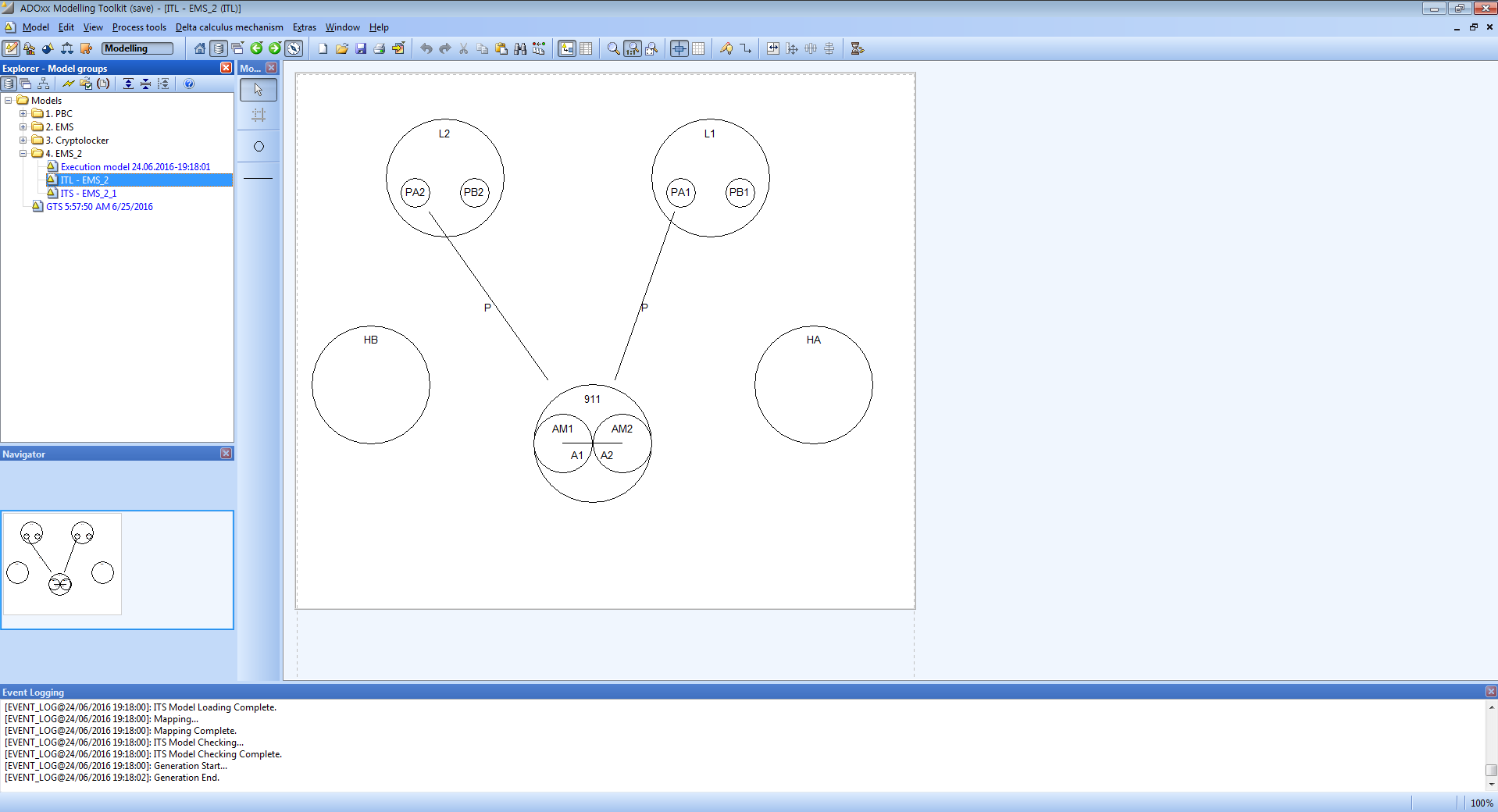5. Step 2: ITS View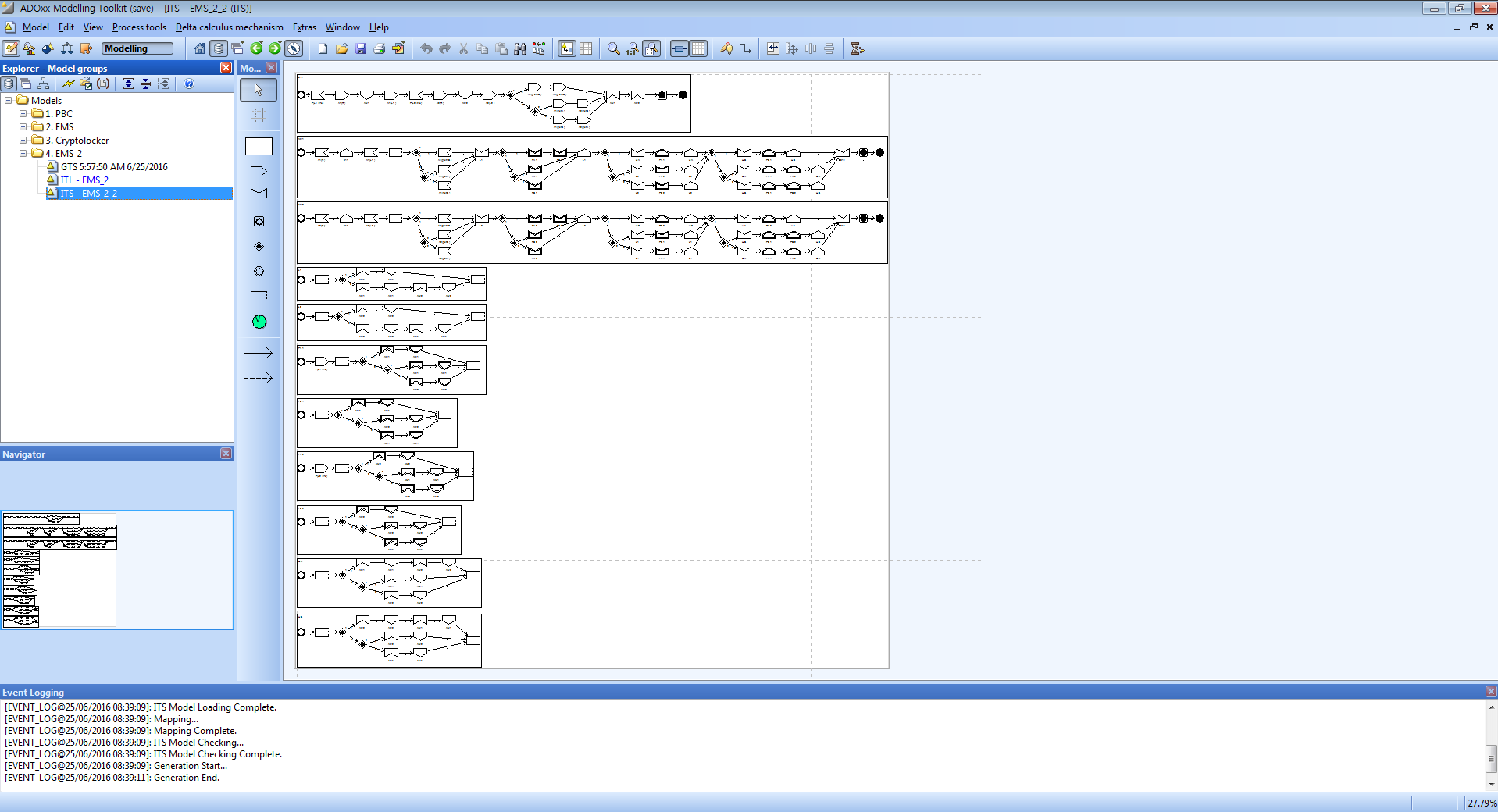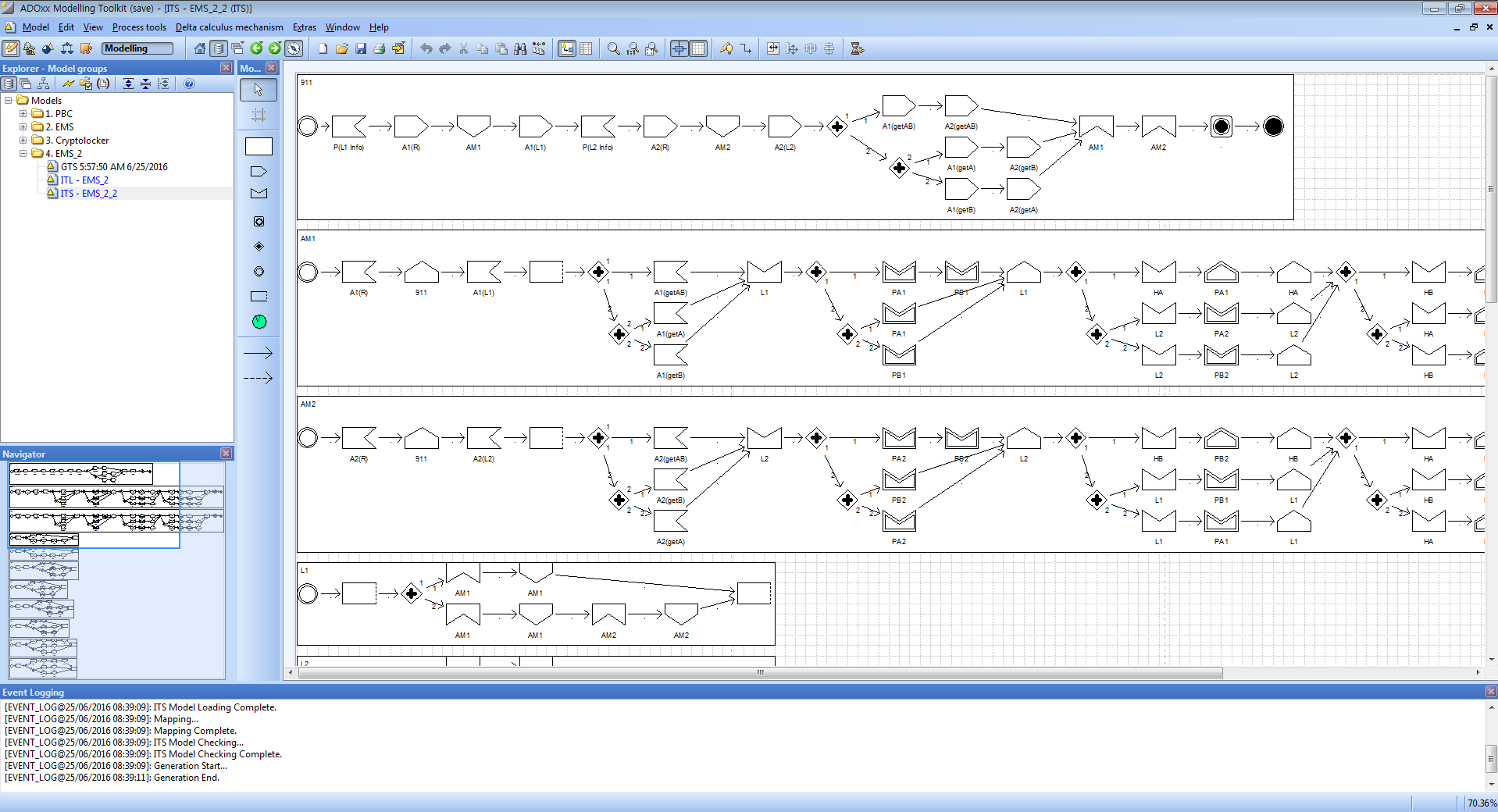6. Step 3: Execution Model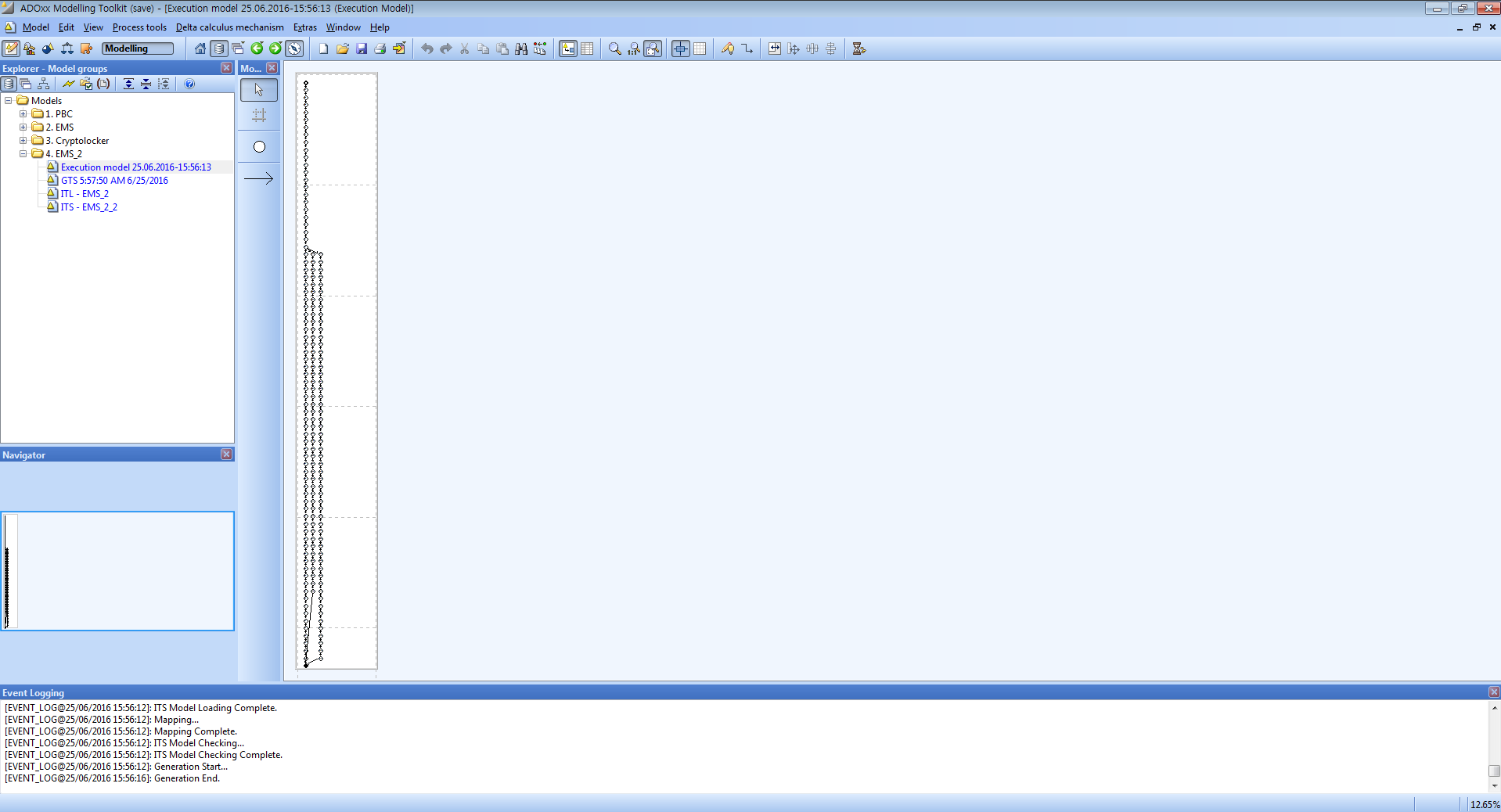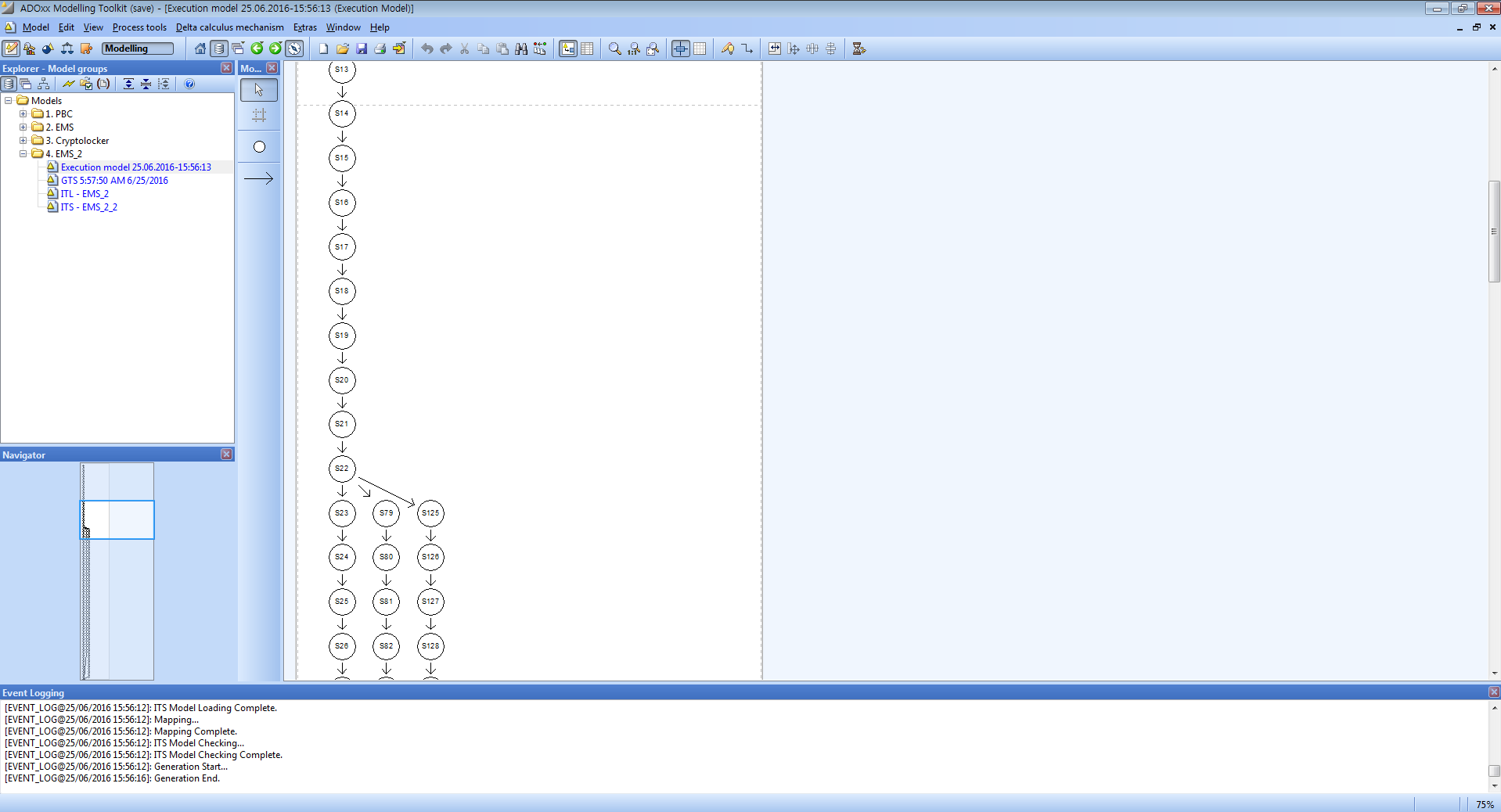7. Simulation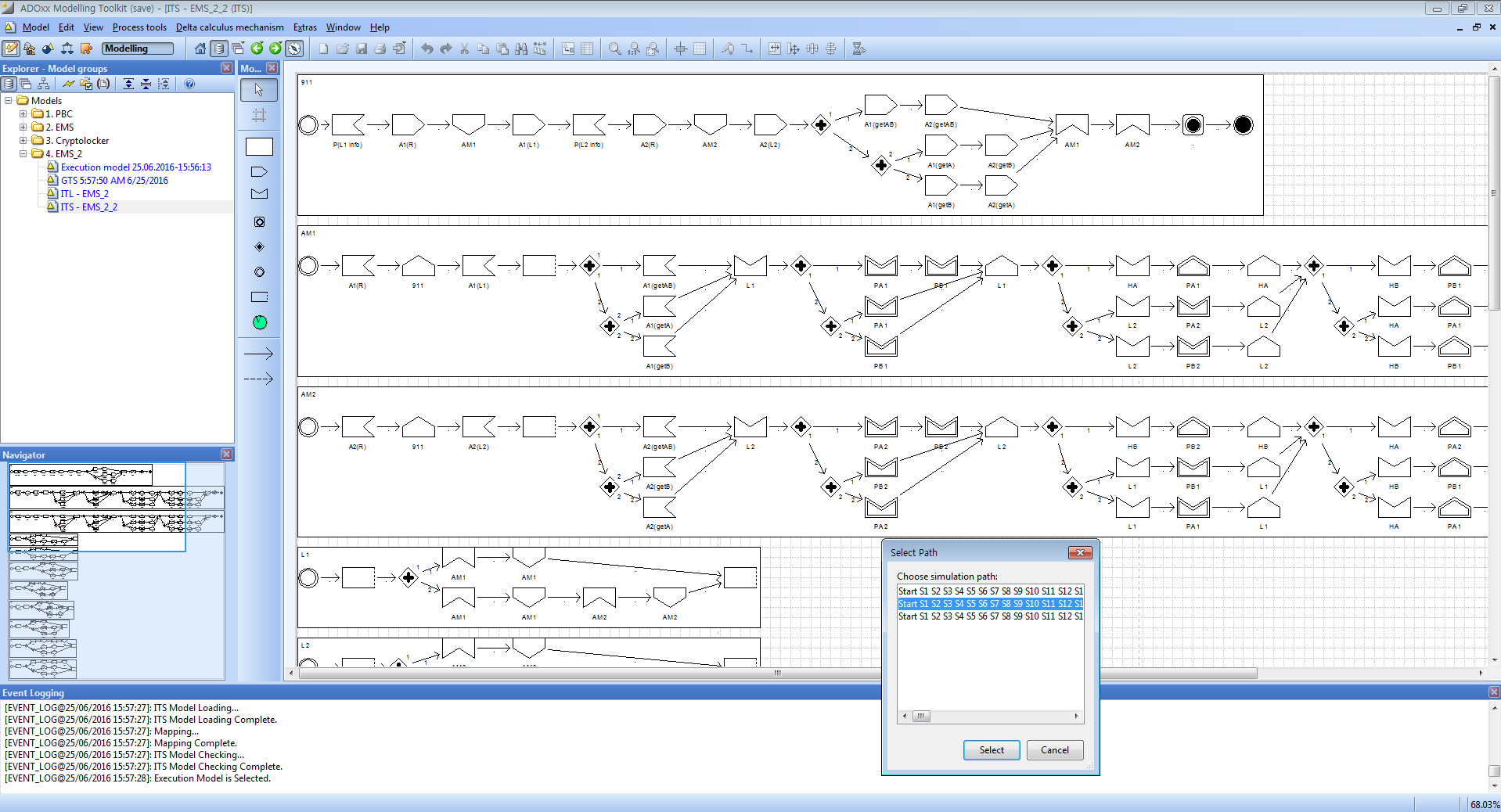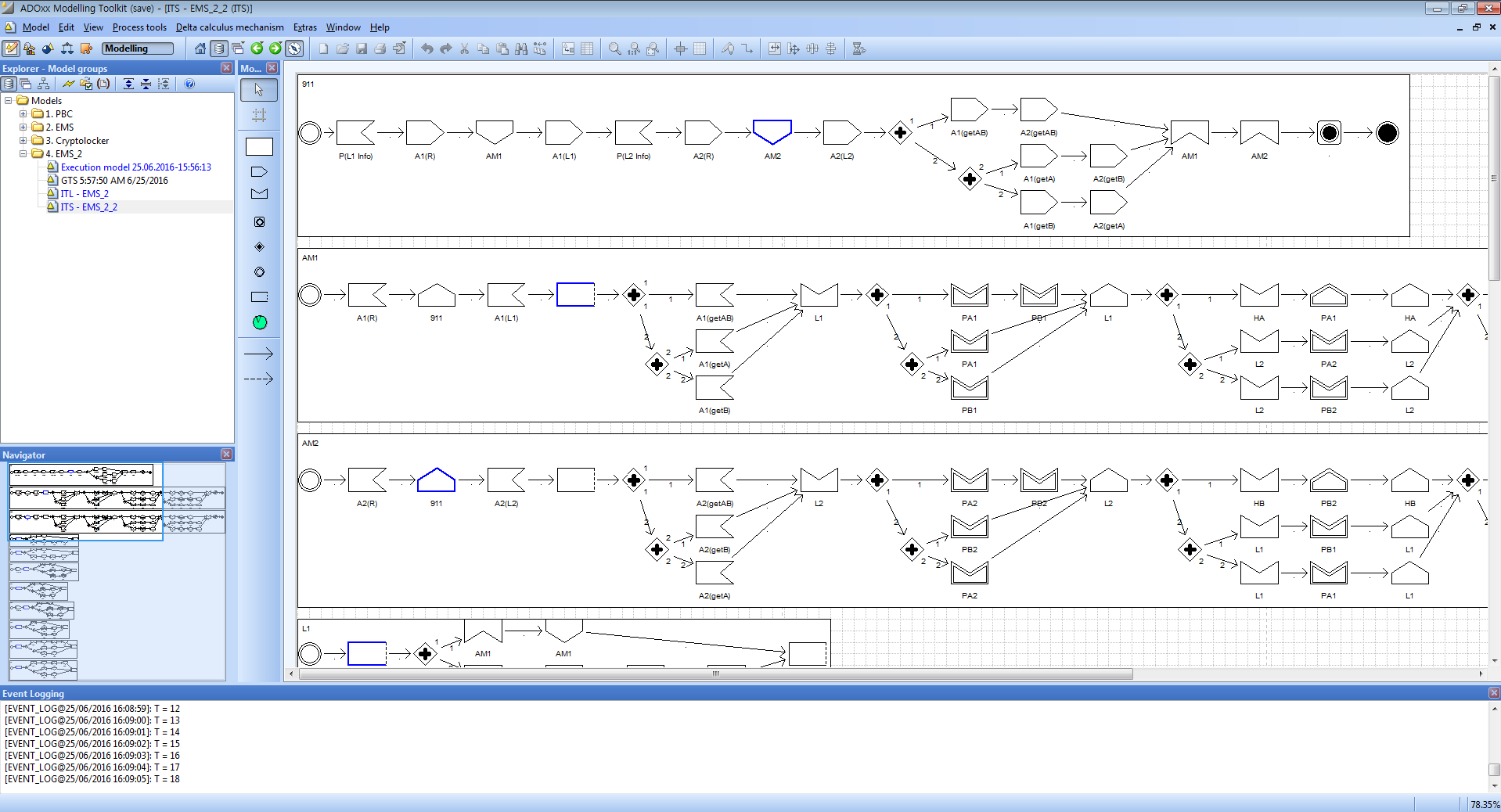8. GTS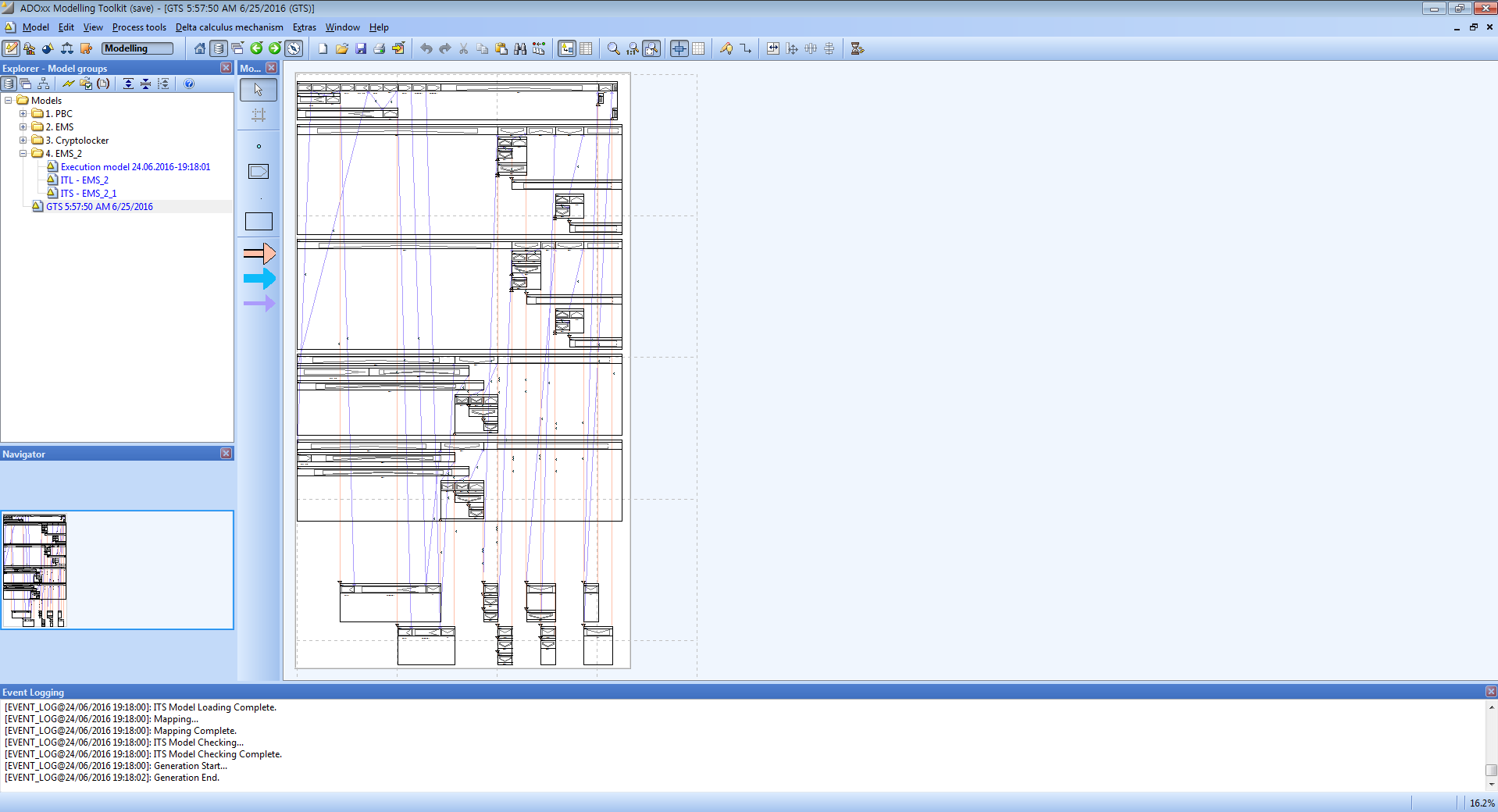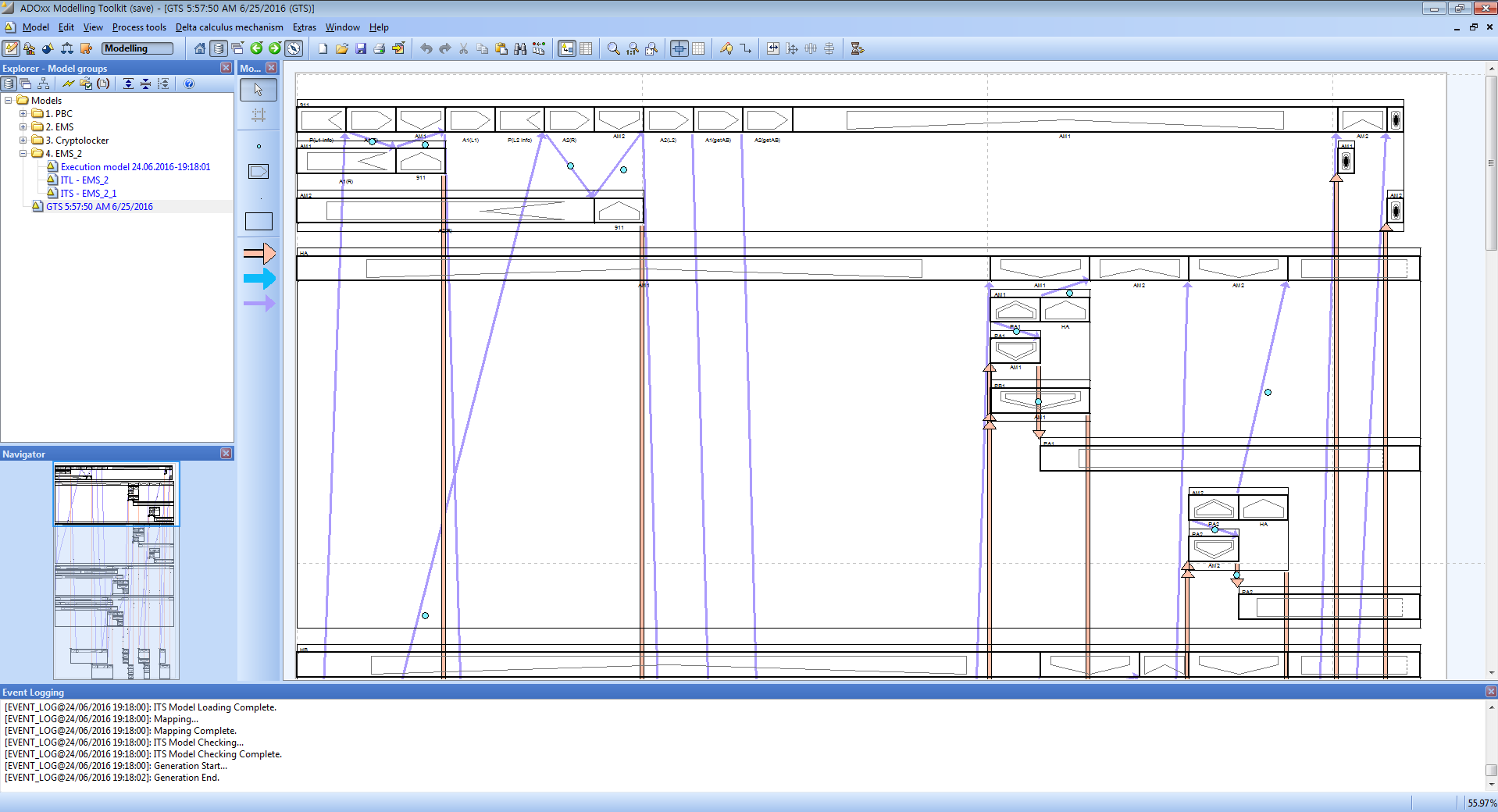9. Secure Requirements

• PA1, PA2 must be transported to HA.
• R1: (AM1:get PA1)→((AM1:in HA)<(AM1:put PA1)<(AM1:out HA))
• R2: (AM2:get PA1)→((AM2:in HA)<(AM2:put PA1)<(AM2:out HA))
• R3: (AM1:get PA2)→((AM1:in HA)<(AM1:put PA2)<(AM2:out HA))
• R4: (AM2:get PA2)→((AM2:in HA)<(AM2:put PA2)<(AM2:out HA))
• PB1, PB2 must be transported to HB.
• R5: (AM1:get PB1)→((AM1:in HB)<(AM1:put PB1)<(AM1:out HB))
• R6: (AM2:get PB1)→((AM2:in HB)<(AM2:put PB1)<(AM2:out HB))
• R7: (AM1:get PB2)→((AM1:in HB)<(AM1:put PB2)<(AM2:out HB))
• R8: (AM2:get PB2)→((AM2:in HB)<(AM2:put PB2)<(AM2:out HB))
• AMB must transport all the patients at one location or of a kind.
• R9: (AM1:get PA1)→((AM1:get PB1)∨(AM1:get PA2))
• R10: (AM1:get PB1)→(AM1:get PB2)
• R11: (AM2:get PA2)→((AM2:get PB2)(AM2:get PA1))
• R12: (AM2:get PB2)→(AM2:get PB1)

10. GTS Logic